21 May 2021

# Motivation

• A unique feature in nonlife insurance risk classification: rating variables are categorical and many have a large number of levels

• The high cardinality in the categorical rating variables imposes challenges in the implementation of the traditional actuarial methods

# Motivation

• A unique feature in nonlife insurance risk classification: rating variables are categorical and many have a large number of levels

• The high cardinality in the categorical rating variables imposes challenges in the implementation of the traditional actuarial methods

• In particular, the generalized linear models (GLMs) have some difficulties

• Unrealistic amount of computational resource due to the high-dimensional design matrix

• The higher likelihood of insufficient data in some categories of the rating variable

• The relationship between different levels of the rating variable is usually ignored

# Goal

We present several actuarial applications of categorical embedding in the context of nonlife insurance risk classification.

• Single insurance risk

• Dependent insurance risks

• Pricing new risks with sparse data

Based on paper:

P. Shi., K. Shi, 2021, Nonlife Insurance Risk Classification Using Categorical Embedding. Available at SSRN.

# What is categorical Embedding?

• The idea is due to Guo and Berkhahn (2016). The method maps each categorical variable into a real-valued representation in the Euclidean space.

• In the embedding space, the categories with similar effects are close to each other. Similar to word embedding in natural languge processing.

# What is categorical Embedding?

• The idea is due to Guo and Berkhahn (2016). The method maps each categorical variable into a real-valued representation in the Euclidean space.

• In the embedding space, the categories with similar effects are close to each other. Similar to word embedding in natural languge processing.

For the categorical variable $$x$$ with $$K$$ levels, the embedding function of $$d$$-dimensional embedding space is given by: \begin{align} e: x \mapsto \bf{\Gamma} \times \bf{\delta}, \end{align}

The $$k$$th category is represented by the $$k$$th column of $$\bf{\Gamma}$$. To see this, for the $$i$$th data point with $$x_i=c_k$$, we note: \begin{align} e(x_i) = \left( \begin{array}{ccc} \gamma_{11} & \cdots & \gamma_{1K} \\ \vdots & \ddots & \vdots \\ \gamma_{d1} & \cdots & \gamma_{dK} \\ \end{array} \right) \times \left( \begin{array}{c} \delta_{x_i,c_1} \\ \vdots \\ \delta_{x_i,c_K} \\ \end{array} \right) = \left( \begin{array}{c} \gamma_{1k} \\ \vdots \\ \gamma_{dk} \\ \end{array} \right). \end{align}

# Categorical Embedding and Nueral Networks

The embeddings can be automatically learned by a neural network in the supervised training process.

• Add an embedding layer, an extra layer between the input layer and the hidden layer, in the neural network

• Treat the embedding matrix as the weight parameters of the embedding neurons# Actuarial Literature

We emphasize that categorical embedding is especially useful in two scenarios:

• It mitigates overfitting and thus leads to better prediction for the neural network.
• Fast growing literautre on applications of neural networks in actuarial applications: Wuthrich and Merz (2019), Wuthrich (2019), Perla et al (2020) among others.
• It is more often that the interest of categorical embedding is the embedding itself rather than the predicted outcome.

# Data

The insurance claims dataset is obtained from the local government property insurance fund of Wisconsin

• We examine the building and contents insurance that covers damage to both physical structures and items inside

• There are over one thousand entities observed during years 2006-2013, resulting in 8,880 policy-year observations.

Description of rating variables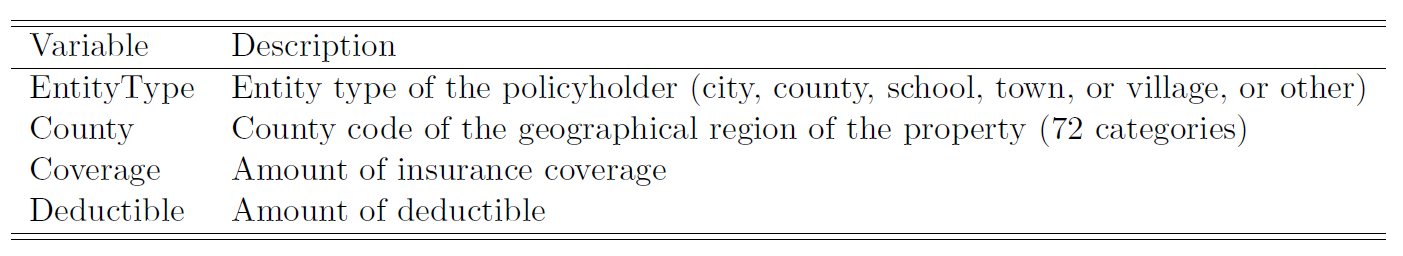# Data

We consider a binary outcome that measures the claim frequency by peril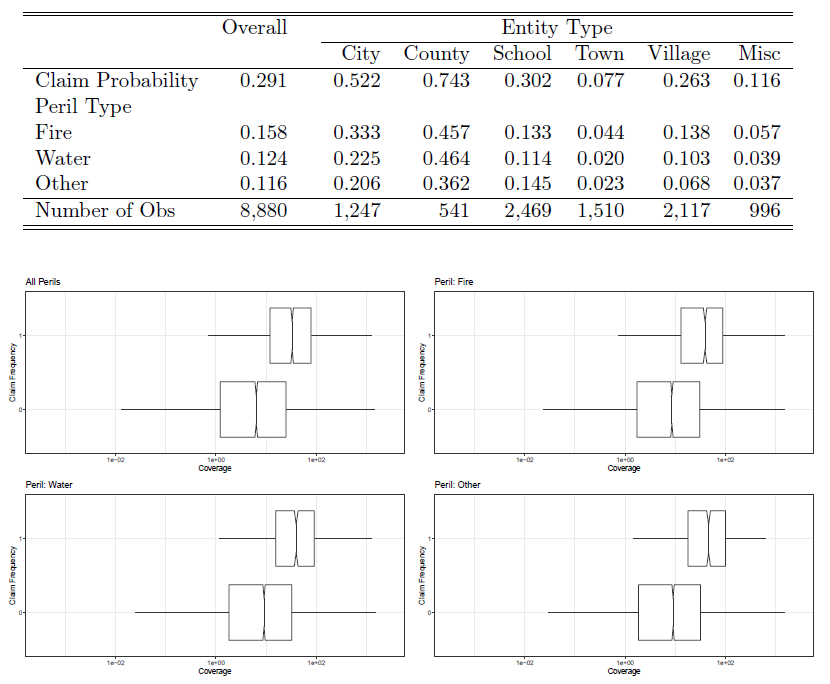# Data

Claim frequency outcomes are dependent: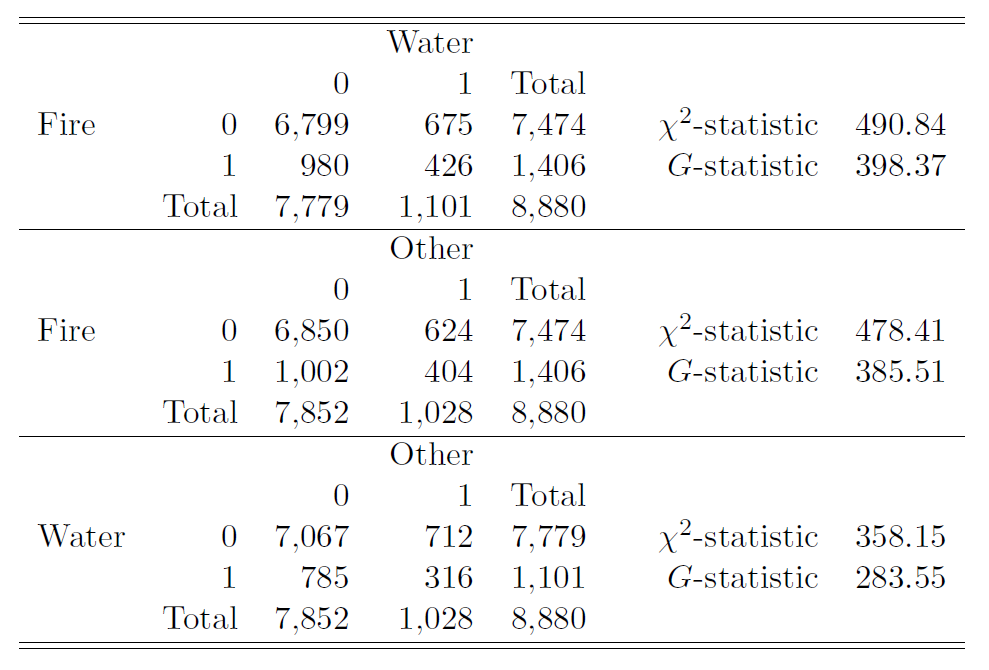# Rating Classes for A Single Risk

In this case, we consider the context where there is a single insurance risk:

• Treat the open-peril property insurance as an umbrella policy

• Define the claim frequency as a risk measurement for the aggregate claims from all peirls

We fit neural networks:

• One-hot encoding

• Categorical embedding

# Rating Classes for A Single Risk

Some results on prediction: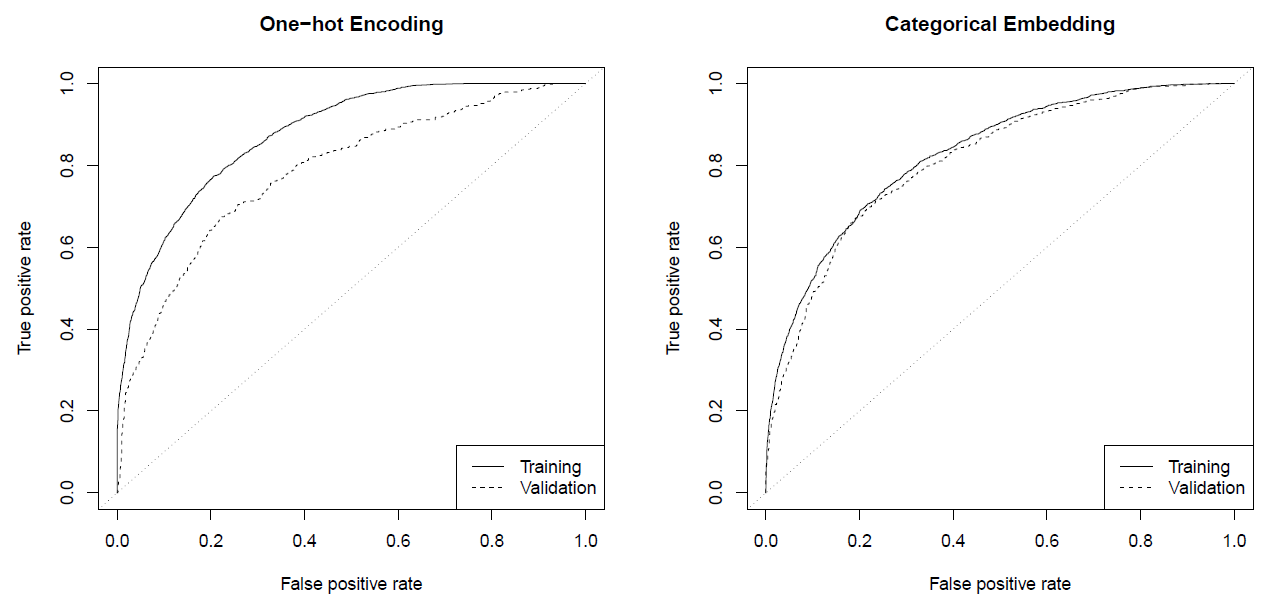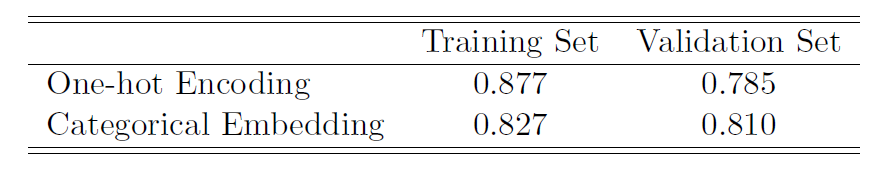# Rating Classes for A Single Risk

We could also use the embeddings to create risk classes: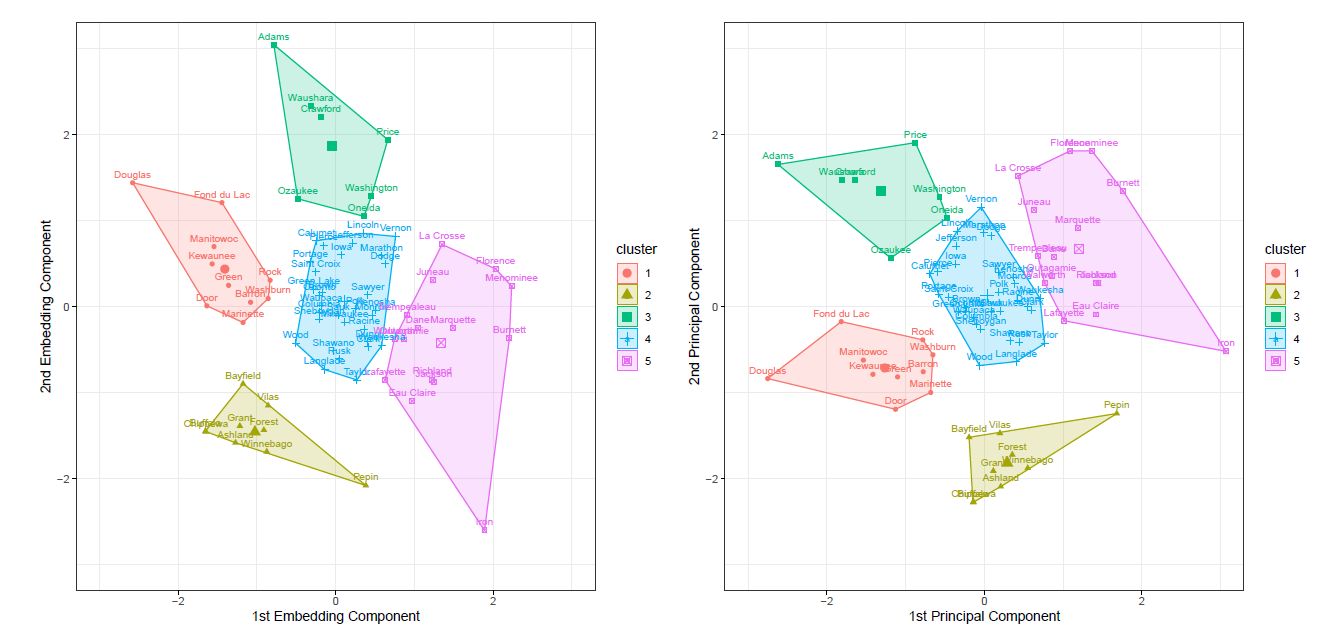# Rating Classes for A Single Risk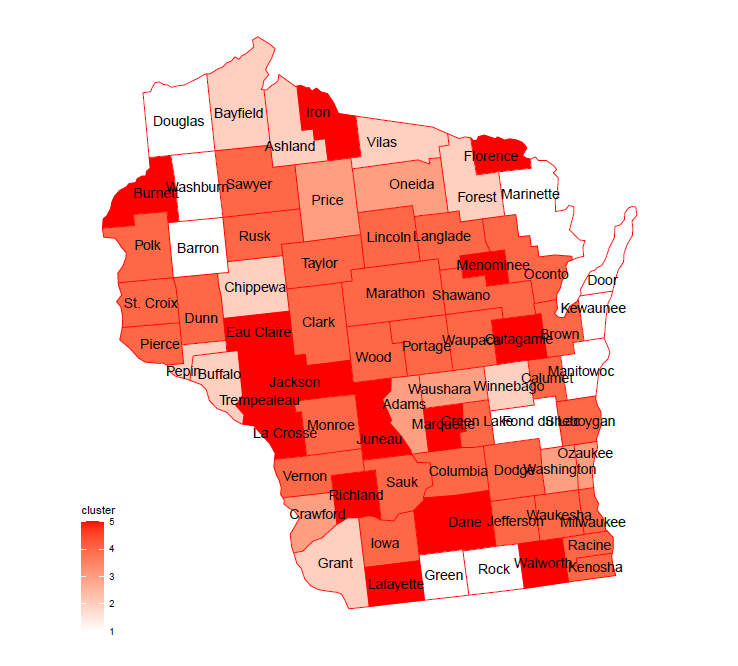# Portfolio Management for Dependent Risks

• In this case, we consider a model for multi-peril risks

• Let $$Z_j$$ be the outcome for peril $$j$$. We formulate the problem as a multi-output network for the vector $$Y=(Z_1,Z_2,Z_3)$$

• We use the dependence ratio to describe the raltionship among perils

\begin{align} \rho(z_1,z_2,z_3) = \frac{{\rm Pr}(Z_1=z_1,Z_2=z_2,Z_3=z_3)}{{\rm Pr}(Z_1=z_1){\rm Pr}(Z_2=z_2){\rm Pr}(Z_3=z_3)} \end{align}

# Portfolio Management for Dependent Risks

• In this case, we consider a model for multi-peril risks

• Let $$Z_j$$ be the outcome for peril $$j$$. We formulate the problem as a multi-output network for the vector $$Y=(Z_1,Z_2,Z_3)$$

• We use the dependence ratio to describe the raltionship among perils

\begin{align} \rho(z_1,z_2,z_3) = \frac{{\rm Pr}(Z_1=z_1,Z_2=z_2,Z_3=z_3)}{{\rm Pr}(Z_1=z_1){\rm Pr}(Z_2=z_2){\rm Pr}(Z_3=z_3)} \end{align}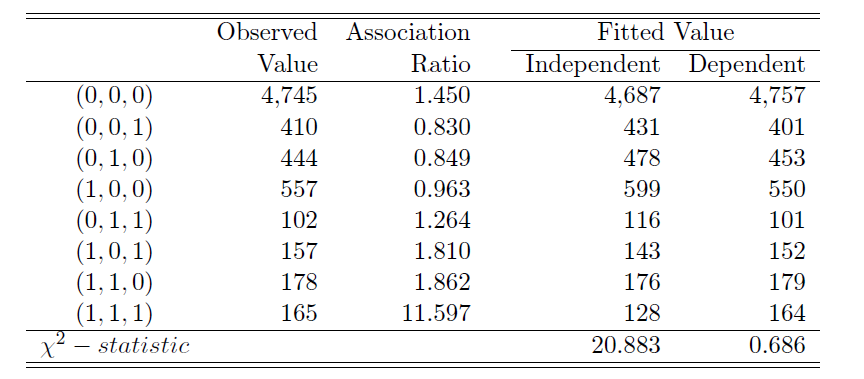# Portfolio Management for Dependent Risks

We consider two types of insurance coverage, the stop-loss insurance and the excess-of-loss insurance. The insurer’s retained loss can be represented as: \begin{align*} {\rm Stop ~loss}:& ~R_1 = \min\{S,d_1\}\\ {\rm Excess~ of~ loss}:& ~R_2 = \max\{S-d_2,0\} \end{align*}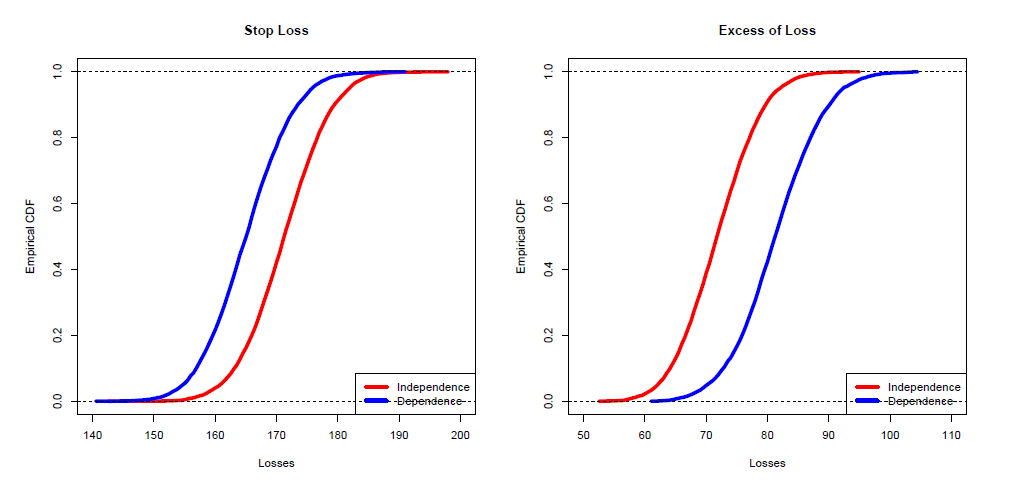# Pricing New Risks

• Suppose that the insurer has only provided coverage for water and other perils during years 2006-2011. Starting from year 2012, the insurer plans to offer fire coverage as well.

• We demonstrate the idea of transfer learning using the categorical variable county.

• Learn the embeddings from single peril: water or other
• Learn the embeedings from the joint bi-peril model: water and other

# Pricing New Risks

• Suppose that the insurer has only provided coverage for water and other perils during years 2006-2011. Starting from year 2012, the insurer plans to offer fire coverage as well.

• We demonstrate the idea of transfer learning using the categorical variable county.

• Learn the embeddings from single peril: water or other
• Learn the embeedings from the joint bi-peril model: water and other
• Comparison of similarity matrix